Latest Banking jobs   »   Quantitative Aptitude Quiz For FCI Phase...

# Quantitative Aptitude Quiz For FCI Phase I 2022- 27th December

Q1. A and B can complete a work alone in 18 days and 24 days respectively. If B started work alone and after 3 days A also joined then in how many days whole work will be completed.
(a) 6 days
(b) 12 days
(c) 8 days
(d) 10 days
(e) 15 days

Q2. A sum on simple interest becomes 7/2 times of itself in ten years, find the rate of interest.
(a) 20%
(b) 16%
(c) 30%
(d) 25%
(e) 12%

Q3. A bag contains 5 blue balls and 7 red balls, if 2 balls are drawn at random. What is the probability that at least 1 ball is red.
(a) 28/33
(b) 17/66
(c) 23/33
(d) 47/66
(e) 27/33

Q4. A train P running with speed 54 kmph crosses a man in 30 sec. In how much time it can cross a 180 m long platform.
(a) 51 sec
(b) 45 sec
(c) 42 sec
(d) 39 sec
(e) 58 sec

Q5. The ratio of milk and water in a vessel is 5 : 8. If 6 liter of milk added in it ratio of milk to water becomes 7 : 8. Find the initial quantity of mixture in the vessel.
(a) 28 liter
(b) 39 liter
(c) 42 liter
(d) 24 liter
(e) 36 liter

Q6. A train crosses a man, who is running in the same direction of train at the speed of 2m/sec. in 10 seconds. The same train crosses a tunnel in 54 seconds. If speed of train is 72 km/h then what is the length of tunnel?
(a) 850 m
(b) 800 m
(c) 900 m
(d) 750 m
(e) 650 m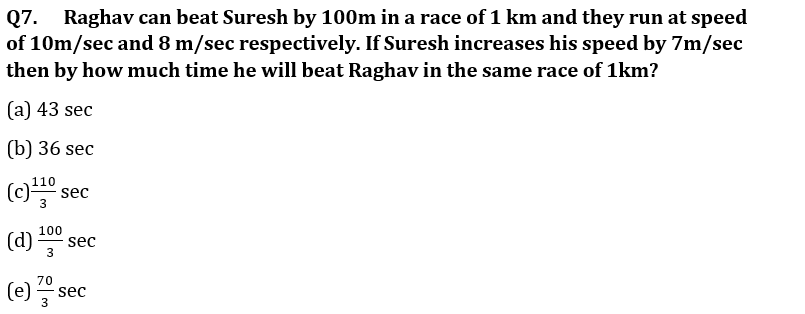Q8. Neeraj and Arun started a business by making investment in the ratio of 3 : 5. After four months, Arun withdraws one fifth of his initial investment. If total annual profit was Rs. 880, find profit share of Neeraj.
(a) Rs. 480
(b) Rs. 360
(c) Rs. 420
(d) Rs. 520
(e) Rs. 320

Q9. In how many different ways the letters of word ‘DANGER’ can be arranged?
(a) 5040
(b) 640
(c) 720
(d)120
(e) 520

Q10. Find the probability of selecting 2 green balls out of 4 green and 3 red balls.
(a) 6/7
(b) 4/7
(c) 3/7
(d) 2/7
(e) 1/7

Q11. The ratio between length and breadth of a rectangular field is 3 : 2. If perimeter of field is 250. Find the area of rectangular field.
(a) 3750 sq. unit
(b) 3570 sq. unit
(c) 3650 sq. unit
(d) 3250 sq. unit
(e) 3950 sq. unit

Q12. A boat can cover upstream distance between two points in 6 hours and same distance downstream in 4 hours. If speed of boat in still water is 8 km/h, then find speed of stream.
(a) 2 km/h
(b) 1.6 km/h
(c) 3.2 km/h
(d) 4.8 km/h
(e) 3.8 km/h

Q13. Two trains X and Y cross each other in 48 sec, when both are running in same direction. If length of train X is 160 m and speed of train X and train Y are 54 km/h and 72 km/h respectively then find the length of train Y.
(a) 160 m
(b) 120 m
(c) 80 m
(d) 100 m
(e) 95 cm

Q14. In a 64 litre mixture of milk and water the ratio of water to milk is 3 : 5. If x litre water is mixed in this mixture, so the ratio of water to milk becomes 5 : 8. Find the value of x.
(a) 6 l
(b) 4 l
(c) 2 l
(d) 1 l
(e) 5 l

Q15. A is 20% more efficient than B who complete the work alone in 20 days. A work first two days alone, then find in how many days remaining work will be completed if both work together?
(a) 6 days
(b) 5 days
(c) 9 days
(d) 8 days
(e) 7 days

Solutions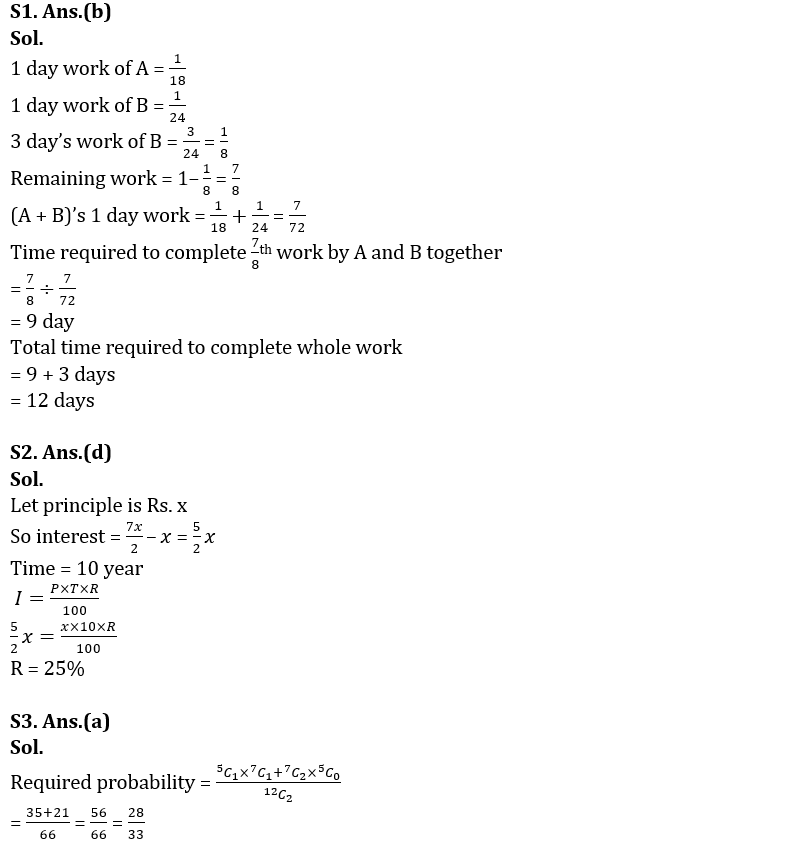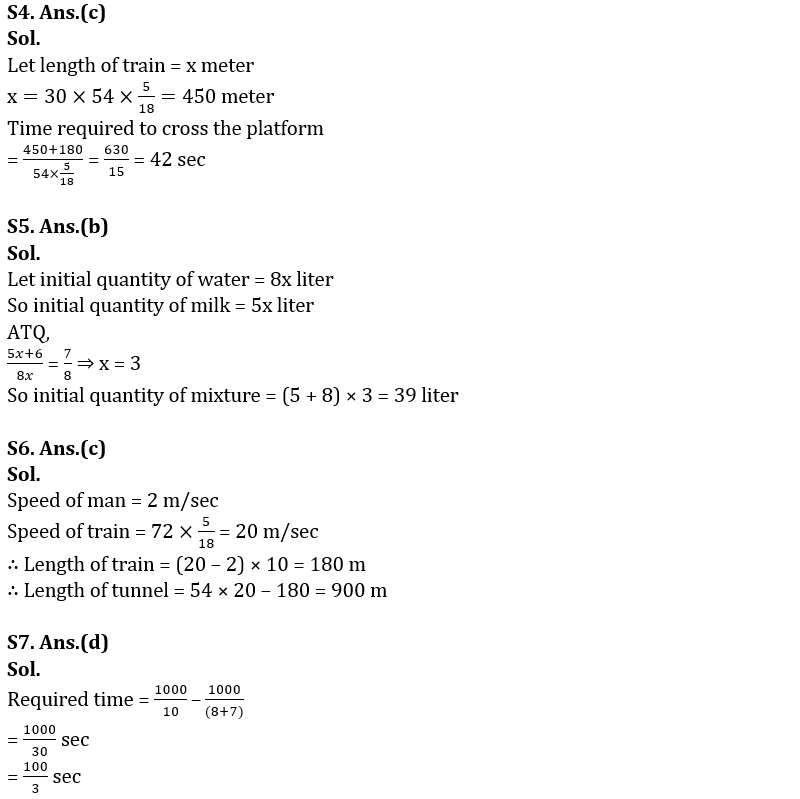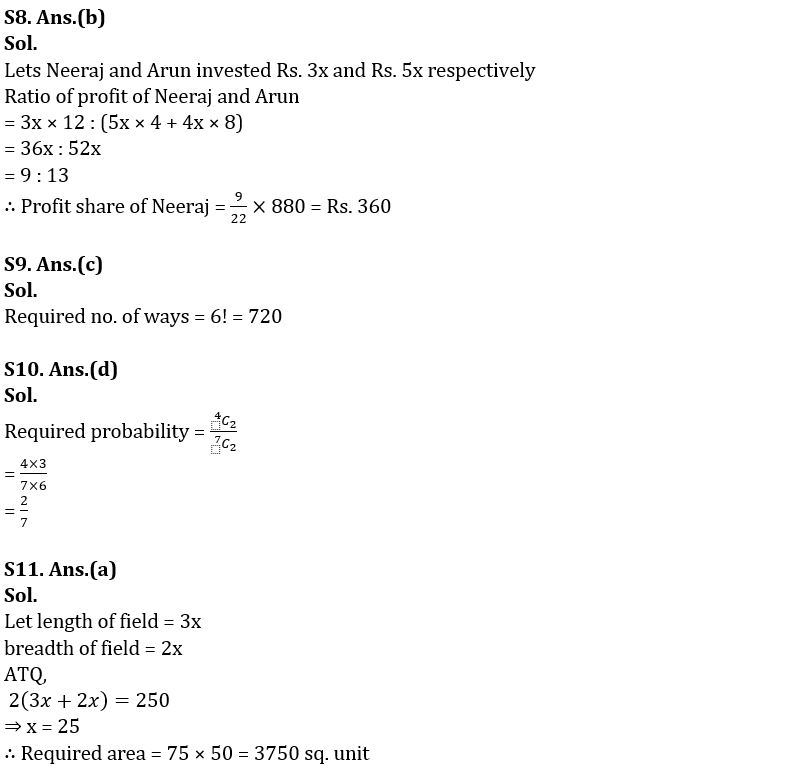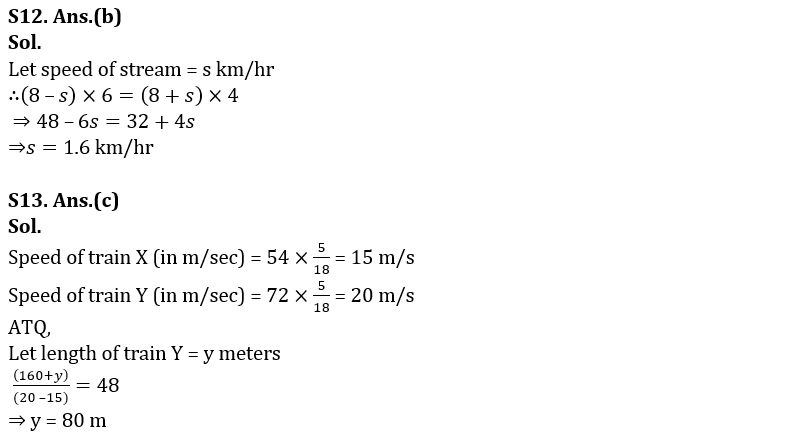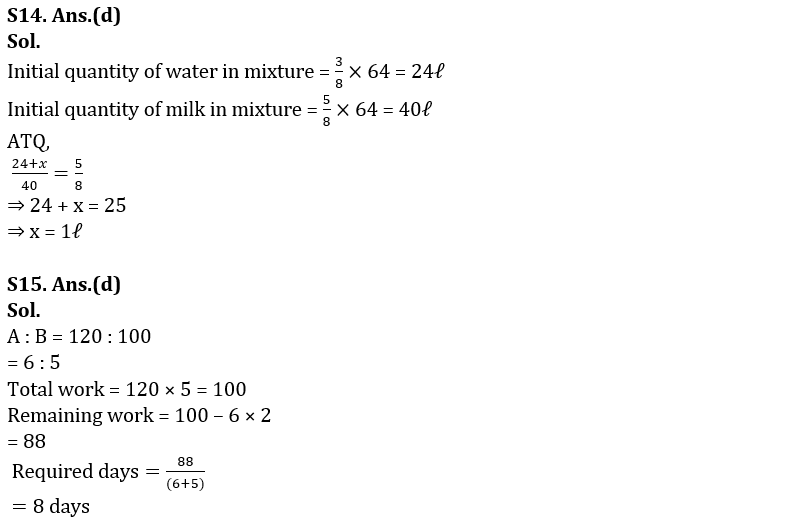#### Congratulations!Union Budget 2023-24: Free PDF